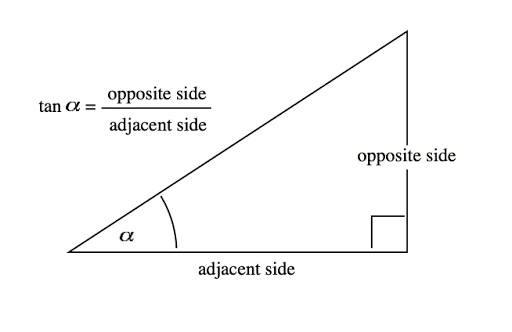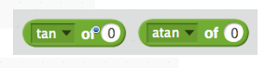## Wednesday, December 23, 2020

### Addendum to Scalene Triangle Mouse I

At the conclusion of the Coding the Scalene Triangle Mouse I document, I described what I considered to be a flaw in the code; when completing the third leg of the triangle the mouse did not actually point to the starting point of the triangle but moved in a skewed fashion.
Scratch does contain a [point towards (mouse-pointer or another sprite)] block.

I suppose one could make the starting point of the triangle a small circular sprite and then simply ‘point to it’ to draw the third leg of the triangle. I call this approach a work-around as it solves the problem for the present but when time allows a more elegant solution will be found. So I thought about how to create code that will point the mouse from one point to any other specified point. In other words, I wanted to code a generalized solution. It turns out that the basic trigonometry operator, tangent (angle) abbreviated tan(angle) helps to solve the problem.In Scratch, the tan operator (see below) returns the numerical ratio of the opposite side over adjacent side as shown in the diagram above.
The arctan operator takes the numerical ratio and returns the angle.
For example, for a 38º angle, tan 38º = 0.7812 and the arctan of 0.7812 = 38ºIn the diagram below the xpos and ypos reporters in Scratch return the values for x2 and y2. The values for x1 and y1 are set in the code. These values are used to compute the tangent of the angle and then the arctangent operator computes the value in degrees of the angle. The mouse can then be turned to the left through the angle (90º – angle)  to be pointed directly towards the point (x1, y1).

I have written a detailed tutorial describing the coding of the above addendum to the Scalene Mouse Triangle project. A free PDF file of this document that might be useful to both teachers and students can be obtained by emailing me at grandadscience@gmail.com.